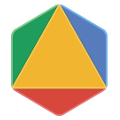OR-Tools Examples

The following table provides links to:

• Code examples in the supported languages:   C++PythonDotNetJava• Tutorials that explain the examples.• Colabs—code demos that you can run directly in your browser.Solver Description Tutorials and colabs Code examples
Routing Simple TSP exampleRouting TSP with distance matrixRouting TSP with 2D locationsRouting Simple Vehicle Routing Problem exampleRouting Vehicle Routing Problem with capacity constraintsRouting Vehicle Routing Problem with pickup & delivery constraintsRouting Vehicle Routing Problem with time window constraintsLinear programming Linear programming hello worldLinear programming Simple linear programLinear programming Integer programming hello worldLinear programming Simple MIP programConstraint programming Constraint programming hello worldConstraint programming Simple CP program